## Polyhedra with Zometool

"Thus, the chief reason for studying regular polyhedra is still the same as in the time of the Pythagoreans, namely, that their symmetrical shapes appeal to one's artistic sense."
H. S. M. Coxeter, in the preface to "Regular Polytopes".

In Figs. 3a and b, we have seen two Zometool models. Such models display only the vertex and edge arrangements of a geometrical object; unlike paper models they cannot display face arrangements. A consequence of this is that the Icosahedron and the Great dodecahedron, which share the same edge arrangements, are represented by the same Zometool model. The same would happen for the Stellated dodecahedron and the Great icosahedron. The three ditrigonal quasi-regular polyhedra in Fig. 7 and the Compound of five cubes in Fig. 8b are all represented by the same Zometool model.

However, this can also be an advantage: because they don't display the faces, they allow the display of internal structure. For the models that follow that is absolutely necessary.

As an example, the first two Zometool models below represent the five Platonic solids. However, they are not represented in isolation (something that would merely repeat the paper models above), but instead in an arrangement that illustrates many interesting geometric relations between them, in a very elegant and economic way. Clearly, such a model cannot be made with paper. The first model in particular was the first Zometool kit I purchased; it was especially useful for me to familiarize myself with the interesting properties of that system, something that I suggest the reader does before attempting to build any of the models that follow.

About these properties: Vertices are represented by connectors (balls), all are identical: they have 12 Pentagonal holes, 20 Triangular holes and 30 rectangular holes, in a pattern that is similar, but not identical, to the faces of the Rhombicosidodecahedron. They are normally provided in white, but Zometool sells them in a variety of colors. All connectors in any Zometool model have the same orientation in space.

Edges are represented by the struts, they are always identical at both ends. Those that connect to the rectangular holes of the balls are in blue (B), Pentagonal holes - red (R), Triangular holes - yellow (Y). Green struts (G) also connect to the Pentagonal holes, but they have a twist that allows them to represent objects with Octahedral symmetry. They also come in half-sizes (HG). All of these come in three regular sizes (0, 1, 2), with distances between the centres of balls attached at both ends multiplied by the Golden Ratio, φ. Red struts also have a very short (00) size. The very large size 3s were made by Zometool in the early days, but they have been discontinued, and for that reason are a bit harder to find. I buy them mostly on eBay.

With that said, let's have a look at the models.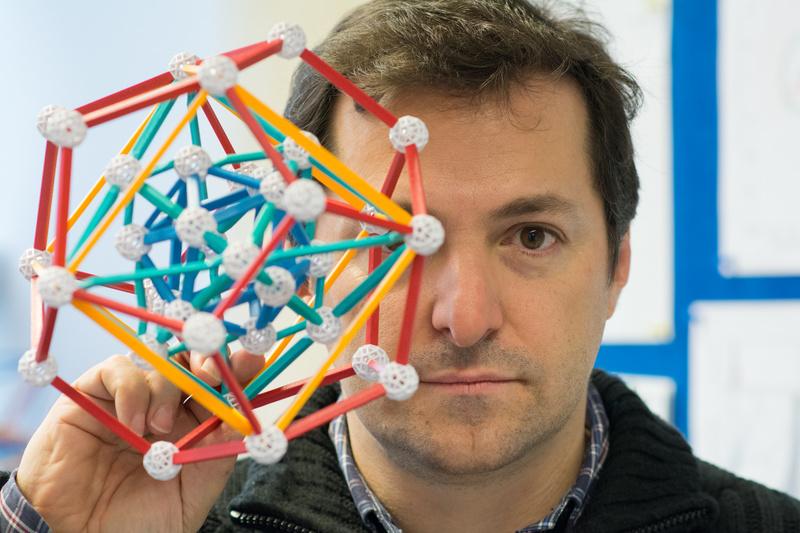Fig. 9a: Myself holding a beautiful geometrical model sold by Zometool as Kepler's Kosmos. Photo by Aris Noutsos.
Note: most of the struts in this and the following model have highly unusual colors, these parts tend to be quite rare. For the subsequent models, I use regular colored parts.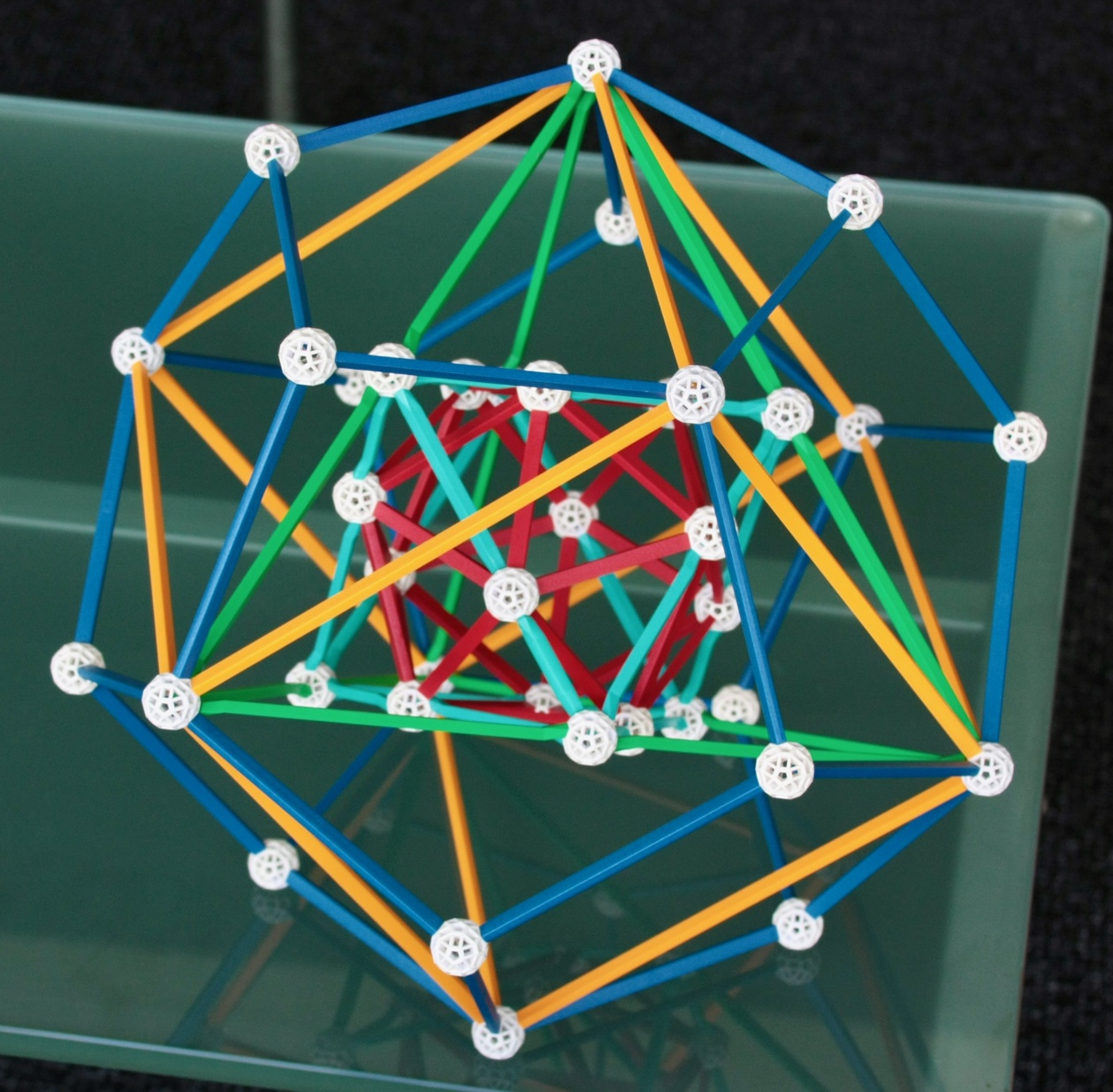Fig. 9b: This is a larger version of the model above, which I have made using some of the specially coloured struts from the model above and some other rare Zometool parts*.

The model in Fig. 9a includes models of the five Platonic solids. This is a kit sold by Zometool with the name ``Kepler's Kosmos''. The arrangement was designed by John H. Conway. Fig. 9b shows a larger version I have built.

The innermost polyhedron, the Icosahedron (represented in the model in Fig. 9b by the red struts) is the dual of the outermost polyhedron, the Dodecahedron (dark blue). The second-innermost polyhedron, the Octahedron (light blue) is the dual of the second outermost one, the Cube (yellow). The middle polyhedron, the Tetrahedron (green) is self-dual. The model is put together in such a way that each vertex of a polyhedron lines up with the face of its dual, and vice-versa; furthermore, the edges of dual polyhedra are perpendicular to each other. Also,
• - The 12 vertices of the Icosahedron touch the 12 edges of the Octahedron.
• △ The 8 faces of this Octahedron are in the same planes as 8 of the 20 faces of the Icosahedron.
• - The 6 vertices of the Octahedron touch, in their midpoints, the 6 edges of the Tetrahedron.
• △ The 4 facial planes of the Tetrahedron coincide with 4 of the 8 facial planes of the Octahedron.
• △ The 4 vertices of this Tetrahedron coincide with 4 of the 8 vertices of the Cube (i.e., the Tetrahedron is "inscribed" in the Cube).
• - The 6 edges of the Tetrahedron lie in the 6 facial planes of the Cube.
• △ The 8 vertices of the Cube coincide with 8 of the 20 vertices of an outer Dodecahedron (i.e., the Cube is inscribed in the Dodecahedron).
• - The 12 edges of the Cube lie in the 12 facial planes of the Dodecahedron.
To summarize: the facial plane coincidences of the inner polyhedra - all of them with triangular faces - are the duals of the vertex coincidences of the outer polyhedra - all of them with triangular vertex figures (see △s). When the vertex of inner polyhedron A touches and edge of inner polyhedron B, the edge of the dual of B lies in the face of the dual of A (see -s).

The construction is possible because the number of vertices of the Octahedron (6) and Icosahedron (12) are the same as the number of edges of the Tetrahedron (6) and Octahedron (12) - by duality, the 6 and 12 edges of the Tetrahedron and Cube correspond to the 6 and 12 faces of the Cube and Dodecahedron.
Challenge to the reader: The numerical coincidence of vertices of the Octahedron and edges of the Tetrahedron is easily explained: the Octahedron is a rectification of the Tetrahedron (see previous page). However, is there any special reason why the number of vertices of the Icosahedron (12) has to be the same as the number of edges of the Octahedron? (An answer is provided in Fig. 18).

The models above have no mirror symmetry; only a type of symmetry called chiral Tetrahedral symmetry. To achieve mirror symmetry, we would need to add a second Tetrahedron, the dual to the one in the model, making a Stella Octangula configuration (Fig. 8a and 10a, b below). Then, we'd have a complete stellation of the Octahedron and a complete faceting of the Cube in this model. The resulting model would then have an overall pyritohedral symmetry.

Now, if we rotate that model four times around an axis going through the centre of two opposite Pentagonal faces of the Dodecahedron, and superpose these to the original - a process we will call here "Quintuplication" - we find that the inner Icosahedron stays unchanged, the Octahedron becomes a Compound of 5 Octahedra (illustrating how it results from a stellation of the Icosahedron), the Stella Octangula becomes a Compound of 10 Tetrahedra (illustrating how the latter compound results from a stellation of the Icosahedron, being self-dual it is also a faceting of the Dodecahedron), the Cube becomes a Compound of 5 Cubes (illustrating how it results from the faceting of a Dodecahedron), and the outer Dodecahedron stays the same. Thus, a 5-fold rotation of an object with pyritohedral symmetry around a carefully chosen axis transforms it into an object with full Icosahedral symmetry.

* Some words on this. The model in 9b represents an improvement compared to the model in Fig. 9a, not only because it is larger, but also because each polyhedron is now represented by a different color: in Fig. 9a, the Octahedron and the Tetrahedron are in the same color, blue-green. That error (later fixed by Zometool) was useful: I used the 12 extremely rare light blue HG2 struts of the Tetrahedron in 9a to build the larger Octahedron in 9b. This is a much improved representation, since its edges are no longer dominated by the twists of the HG parts. The inner Icosahedron in 9b is made with the 30 rare red B1 struts of the outer Dodecahedron in 9a. The outer Dodecahedron in 9b is made using commonplace B2 struts, here in a darker color. For the Cube in 9b, I've had for a long time the rare yellow B3 equivalents of the B2 struts in 9a; what finally made the model in 9b possible was the surprise inclusion of a set of extremely rare HG3 struts (in their original color, green) in a large lot of Zometool parts I have purchased in 2019, they are used in the Tetrahedron in 9b.

I like the color scheme of the model in Fig. 9b very much. The colors of the dual pairs are opposed: If we associate these colors with a sequence in the Rainbow (red, yellow, green, blue-green, dark blue/violet), then the inner/outer solids have the extremes of the sequence, the middle solid has the middle color (green) and the others have the remaining, not-so-extreme colors. Relative to Fig. 9a, the colors of the inner/outer pair were exchanged, something that increases the contrast between polyhedra and makes it easier to distinguish them.

***

We now show some models representing stellations of the five convex isohedral polyhedra in Figs. 2a,b and 4 that can be stellated. This set consists of 3 Platonic polyhedra (the Octahedron, Dodecahedron and Icosahedron) plus the Rhombic dodecahedron and Rhombic triacontahedron. Being isohedral, they have only one stellation diagram; this makes the model much easier to plan and results in more appealing models. We also present facetings of the Cube, Icosahedron and Dodecahedron; however the latter models are not permanently in my office for lack of space. Among these stellations and facetings we meet many objects that are already familiar, almost all of them were represented above as paper models.

Note: The models with Icosahedral symmetry use size 3 struts.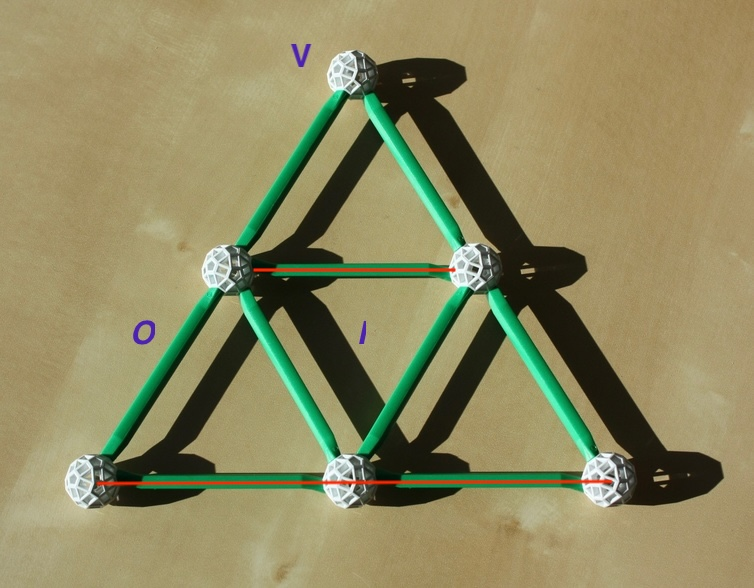Fig. 10a: This is the stellation pattern of the Octahedron. The inner Triangle is the face of the Octahedron. The outer dual Triangle, with an edge that is twice as long as that of the inner Triangle, is the face of its only stellation, the Compound of two tetrahedra (Fig. 8a). This type of stellation is called a "greatening".Fig. 10b: Expanding all faces of the Octahedron as in the previous Figure, we obtain a model of the Octahedron and the Compound of two tetrahedra. Photo by Aris Noutsos.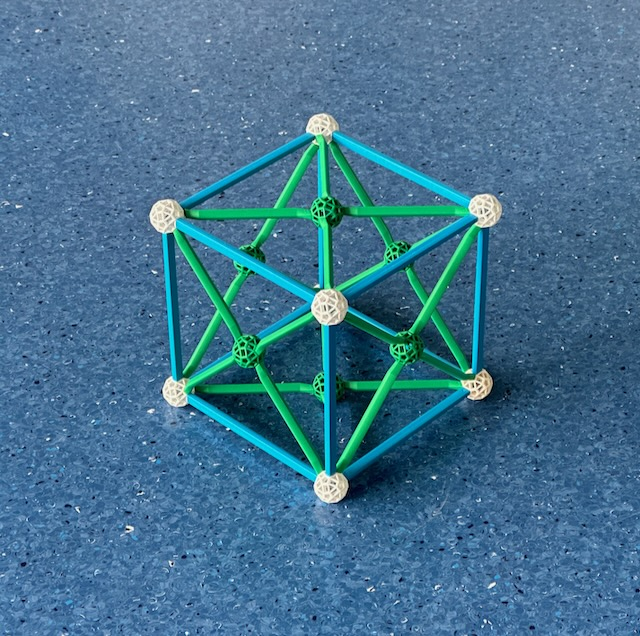Fig. 10c: The dual of the previous model, showing the facetings of the Cube, which appears outside, its vertices (in white) are the only real vertices in the model. All green balls represent false vertices, where edges intersect but don't end. Further in, with the same vertex arrangement of the Cube, is the Compound of two tetrahedra, this also appears here because it is a self-dual compound.Fig. 11a: This is the stellation pattern of the Dodecahedron; its face is the inner Pentagon. The inner Pentagram, with edge length φ3 that of the inner Pentagon, is the face of the Stellated dodecahedron. The outer Pentagon - a φ2 larger dual of the inner Pentagon - is the face of the Great dodecahedron, and the outer Pentagram - a φ2 larger dual of the inner Pentagram - is the face of the Great stellated dodecahedron.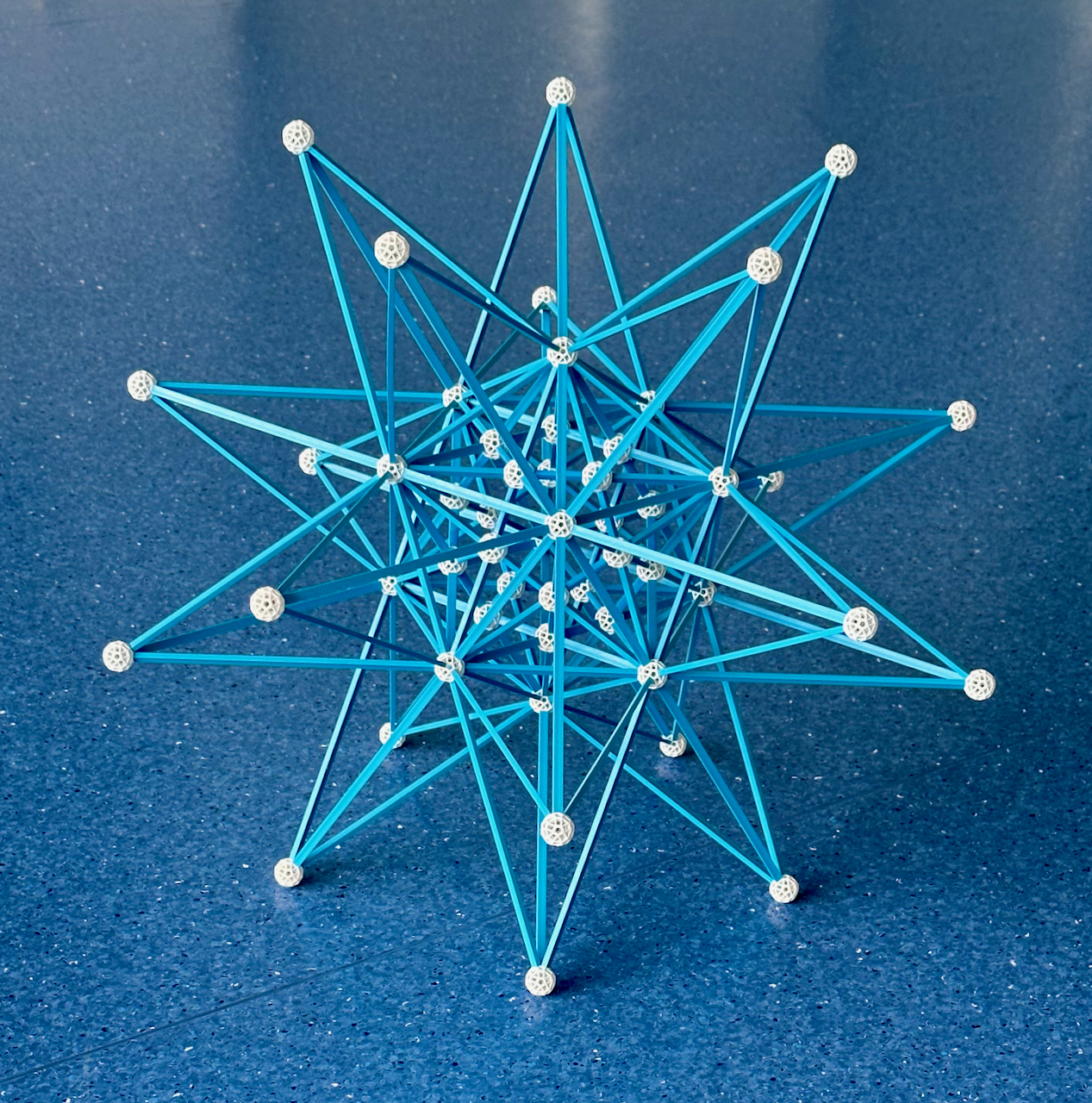Fig. 11b: Extending the 12 faces of the Dodecahedron as in Fig. 11a, we obtain the four stellations of the Dodecahedron (see Fig. 5, and blue and green rectangle in Diagram Ib).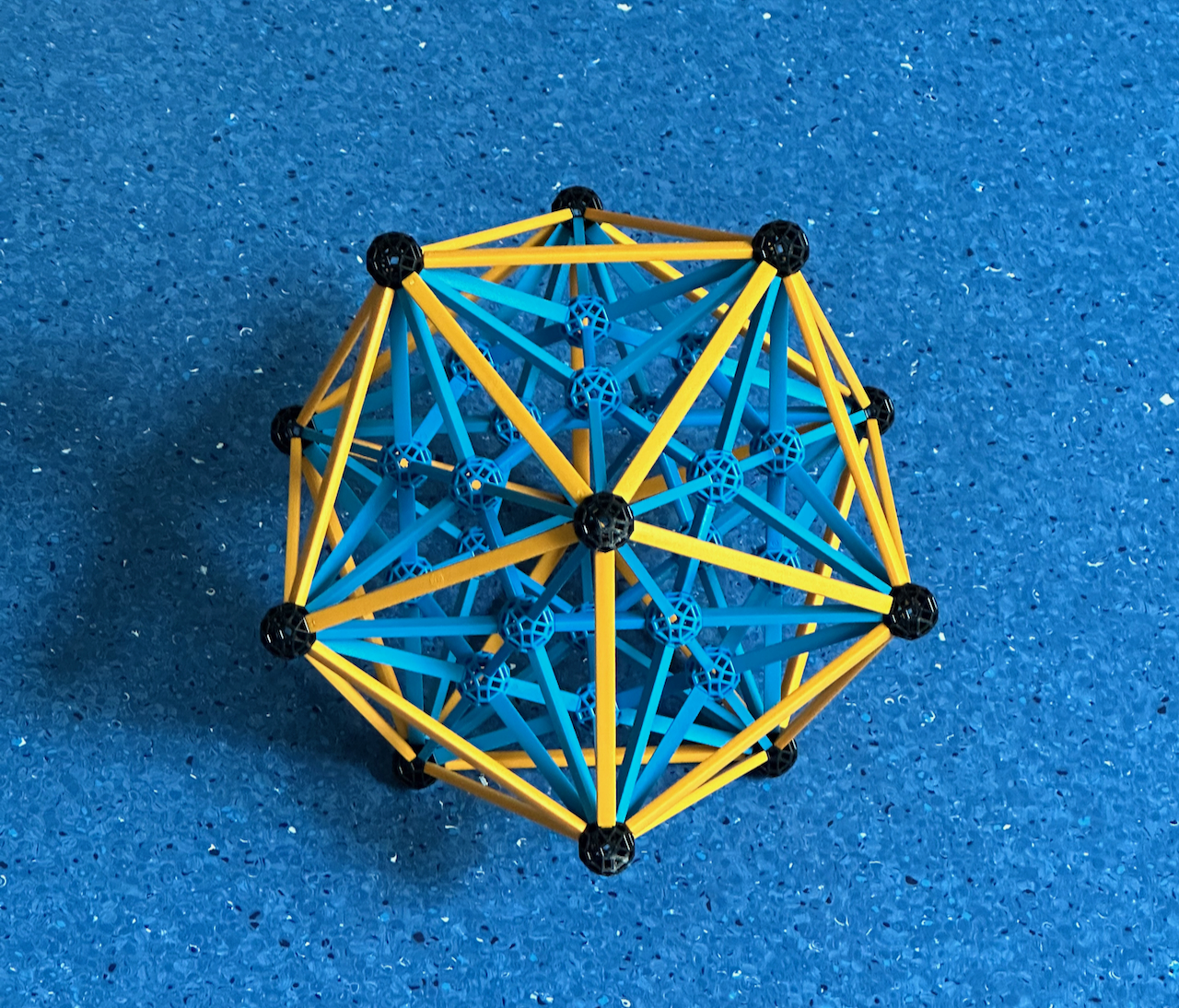Fig. 11c: In this model, the dual of the model in Fig. 11b, we represent the four facetings of the Icosahedron (see Fig. 5 and red rectangle in Diagram Ib). The outer polyhedron is the Icosahedron, this contains all the true vertices of the model (in white). All green balls represent false vertices, where edges intersect but don't end. Further in, with the same vertex and edge arrangement but pentagonal faces, is the Great dodecahedron. Further in, with the same vertex figure of the Icosahedron, is the Stellated dodecahedron. Finally, the innermost polyhedron, with the same edge and vertex arrangement of the Stellated dodecahedron but with triangular faces is the Great icosahedron. The model has the same appearance as the model in Fig. 11b, except for the absence of the outer layer.

This model has been disassembled.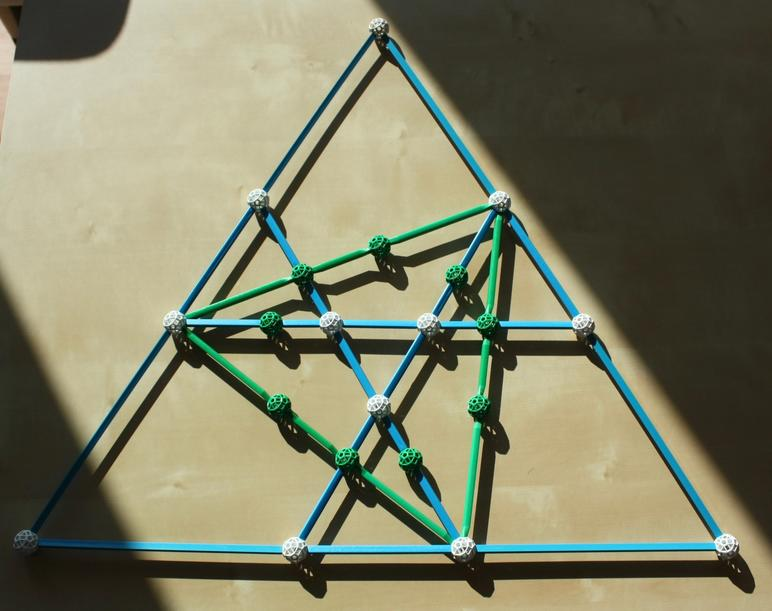Fig. 12a: An incomplete stellation diagram of the Icosahedron, representing some of the figures that can be built with a small Zometool model. The inner blue Triangle is the face of the Icosahedron, the green Triangle is a face of the Compound of five tetrahedra (see Fig. 8a) and the outer φ4 larger dual Triangle is a face of the Great icosahedron (see Fig. 5). An intermediate figure where each face is composed of three disjointed (blue) triangles, touching the face of the Icosahedron at its vertex, is the self-dual Excavated dodecahedron, also known as Deltahedron (which is, therefore, another faceting of the Dodecahedron). These are only four of the 59 stellations of the Icosahedron, some others were presented already in Fig 6.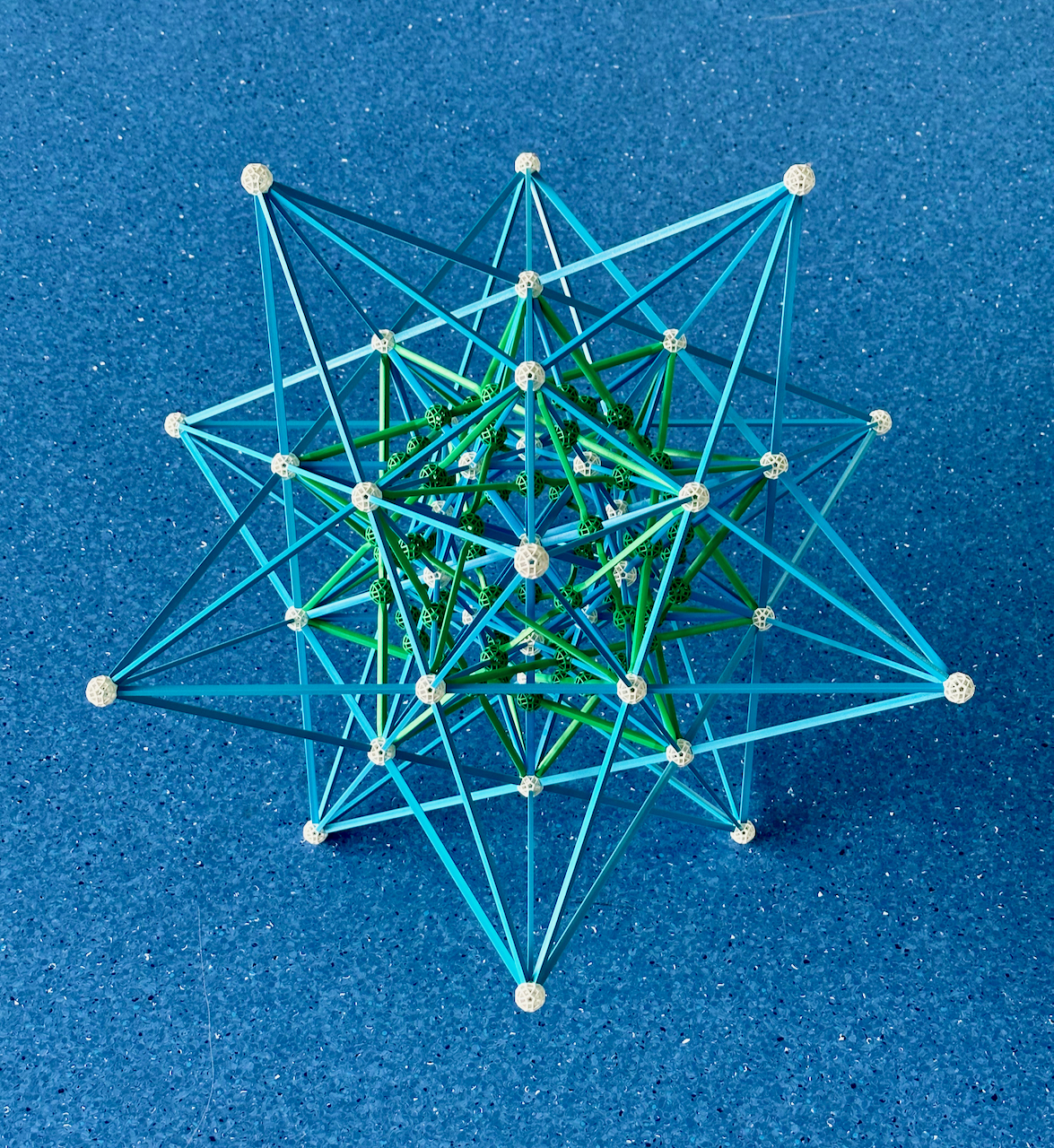Fig. 12b: Extending the 20 faces of the Icosahedron as shown above, we have the four stellations of the Icosahedron mentioned above.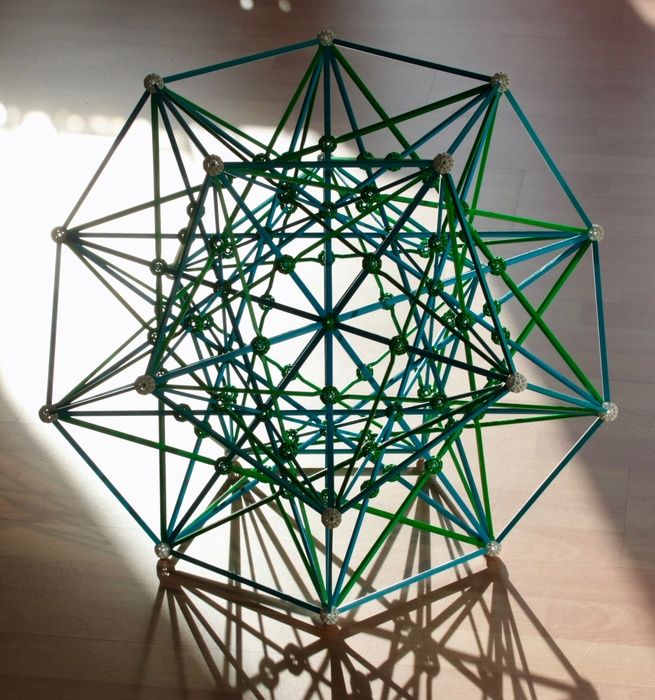Fig. 12c: In this model, the dual of the model in Fig. 12b, we represent four of the many facetings of the Dodecahedron. The outer polyhedron is the Dodecahedron, this contains all the true vertices of the model (in white). All green balls represent false vertices, where edges intersect but don't end. Further in is the Excavated dodecahedron, also present here because it is self-dual. Further in is the Compound of five tetrahedra, also self-dual. Finally, the innermost polyhedron is the Great stellated dodecahedron (see Fig. 5). The model has the same appearance as the model in 12b, except for the absence of the outer layer.

We now show some stellations of the rhombic polyhedra, but not of their duals (they cannot be built with Zometool at a convenient scale).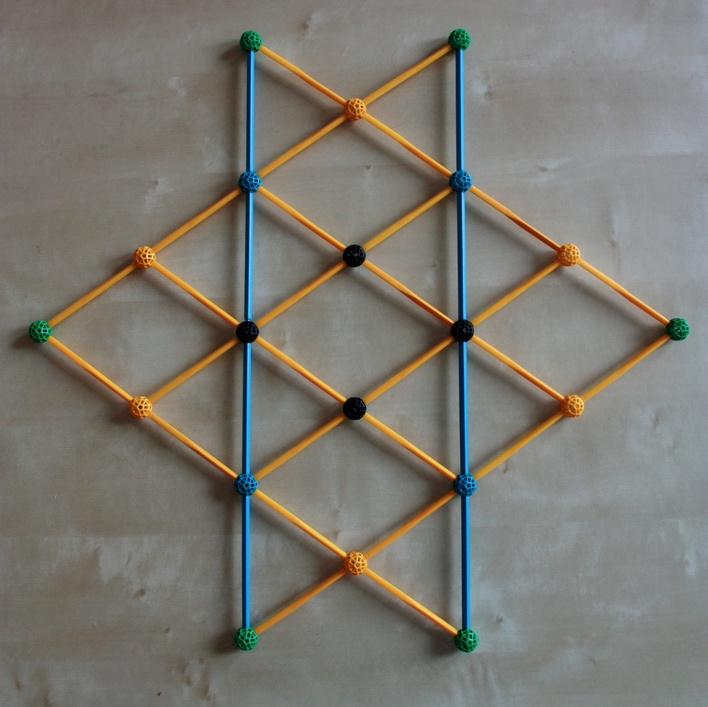Fig. 13a: The stellation diagram of the Rhombic dodecahedron built with Zometool. The inner diamond, with black vertices, is the face of the Rhombic dodecahedron. The first stellation has blue vertices, the second yellow vertices and the final stellation has green vertices.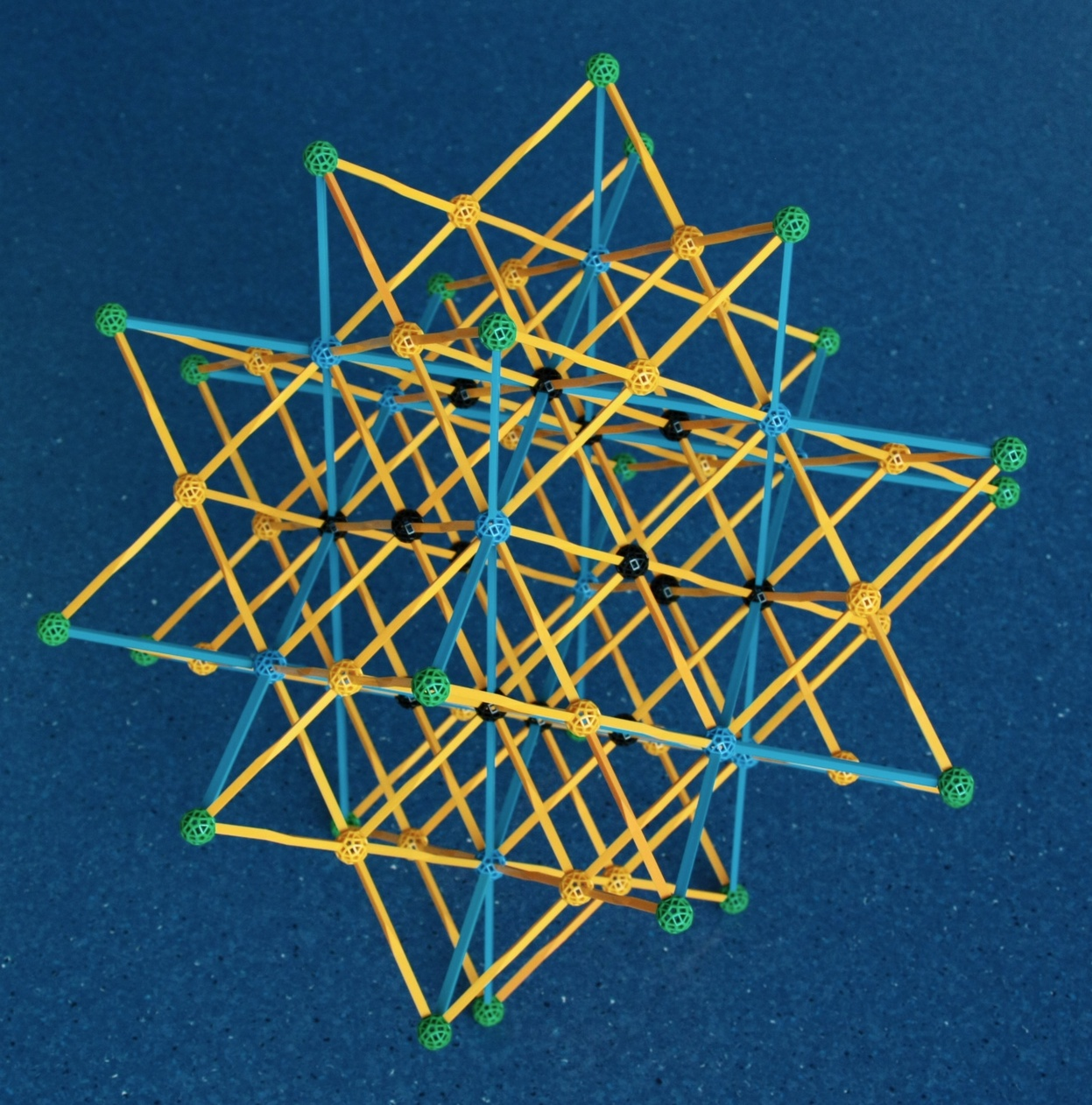Fig. 13b: The four stellations of the Rhombic dodecahedron. Each of the 12 faces of the Rhombic dodecahedron has been extended along their planes as shown above. The different ball colours show the vertices of successive stellations.

Below, we show some of the many stellations of the rhombic triacontahedron. For more on them and instructions on how to build them, look here.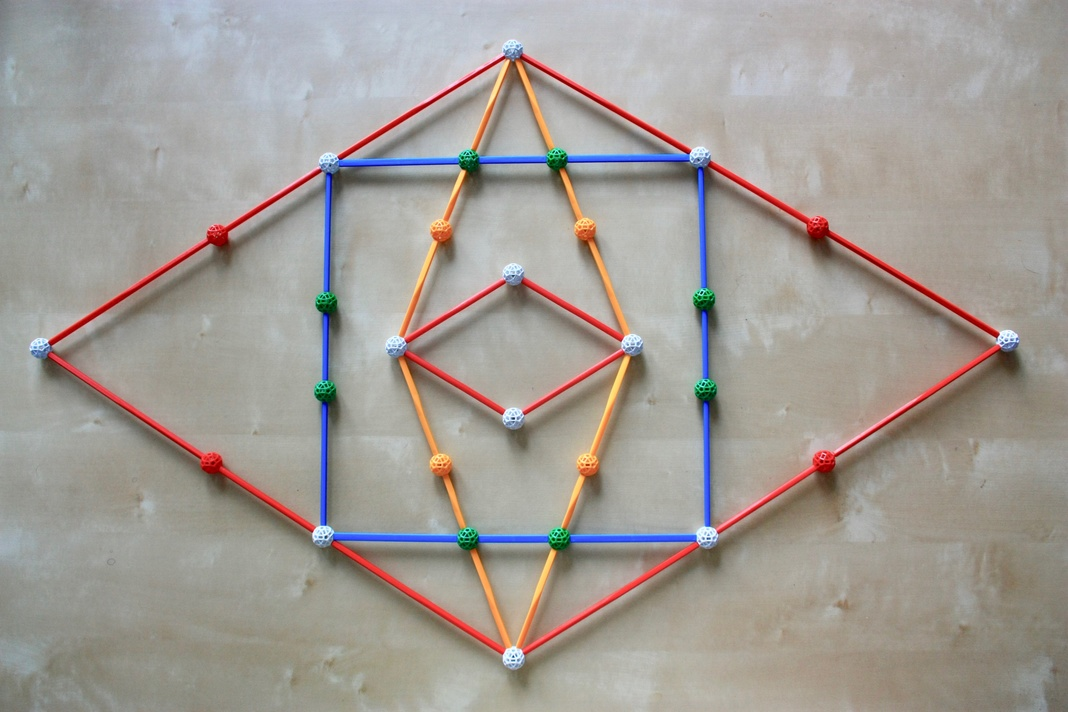Fig. 14a: Within the inner region of the stellation diagram of the Rhombic triacontahedron (Fig. 4), we can find its four isotoxal stellations, for which we have built paper models above. The inner red Rhombus is one of the 30 faces of the Rhombic triacontahedron itself. The yellow Rhombus is one of the 30 faces of the Medial rhombic triacontahedron (see Fig. 6a). The blue Square is one of the 30 faces of the Compound of 5 cubes (Fig. 8b). The outer Rhombus is one of the 30 faces of the Great rhombic triacontahedron (Fig. 6a).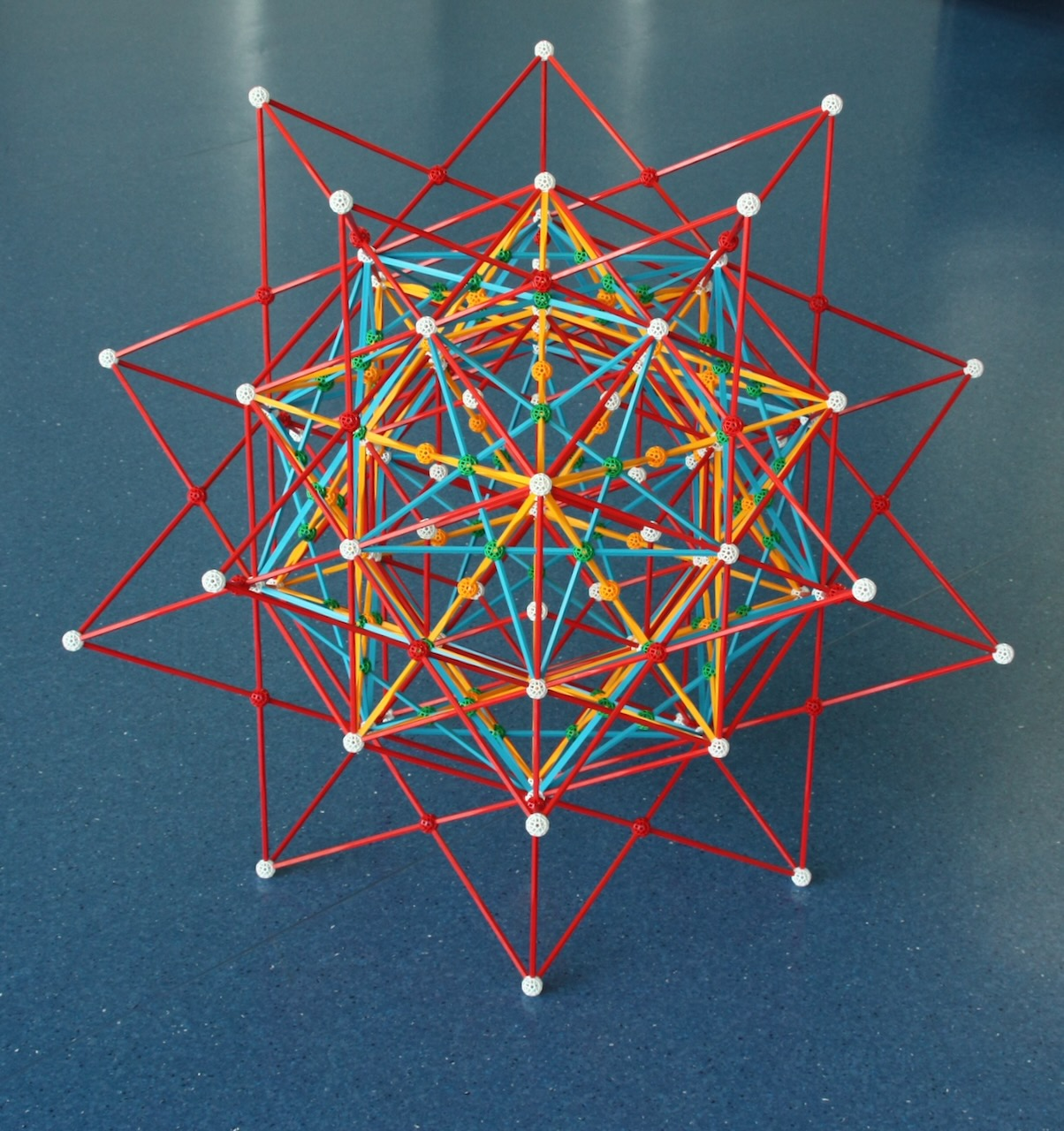Fig. 14b: Extending the 30 faces of the Rhombic triacontahedron as in Fig. 14b, we obtain this model. The four stellations can be found very easily since they are represented by different colours.

One last word about the models in this page. They are the closest in the whole site to artistic objects: one can choose which combinations of polyhedra do show, and their sizes and orientations relative to each other. The models in Figs. 9a and b, by John Conway, are truly beautiful and elegant works of art. Regarding the other models, although they merely display stellations and facetings, they can also be very pleasing. I am particularly happy with those in Figs. 12b and 14b.

Main polytope page. Next: Polychora